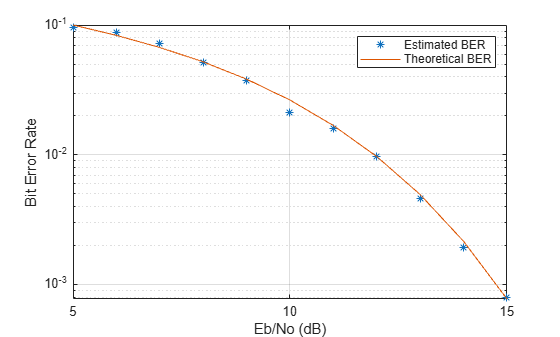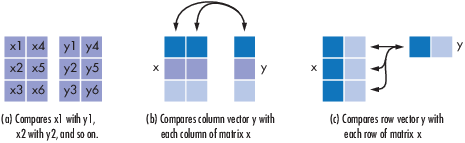# biterr

Number of bit errors and bit error rate (BER)

## Syntax

``[number,ratio] = biterr(x,y)``
``[number,ratio] = biterr(x,y,k)``
``[number,ratio] = biterr(x,y,k,flag)``
``[number,ratio,individual] = biterr(___)``

## Description

example

````[number,ratio] = biterr(x,y)` compares the unsigned binary representation of elements in `x` to those in `y`. The function returns `number`, the number of bits that differ in the comparison, and `ratio`, the ratio of `number` to the total number of bits. The function determines the order in which it compares `x` and `y` based on their sizes. For more details, see Algorithms section.```
````[number,ratio] = biterr(x,y,k)` also specifies `k`, the maximum number of bits for each element in `x` and `y`. If the unsigned binary representation of any element in `x` or `y` is more than `k` digits, the function errors.```

example

````[number,ratio] = biterr(x,y,k,flag)` specifies a `flag` to override default settings for how the function compares the elements and computes the outputs. For more information, see Algorithms section.```
````[number,ratio,individual] = biterr(___)` returns the binary comparison result of `x` and `y` as matrix `individual`. You can specify any of the input argument combination from the previous syntaxes.```

## Examples

collapse all

Create two binary matrices.

`x = [0 0; 0 0; 0 0; 0 0]`
```x = 4×2 0 0 0 0 0 0 0 0 ```
`y = [0 0; 0 0; 0 0; 1 1]`
```y = 4×2 0 0 0 0 0 0 1 1 ```

Determine the number of bit errors.

`numerrs = biterr(x,y)`
```numerrs = 2 ```

Compute the number of column-wise errors .

`numerrs = biterr(x,y,[],'column-wise')`
```numerrs = 1×2 1 1 ```

Compute the number of row-wise errors.

`numerrs = biterr(x,y,[],'row-wise')`
```numerrs = 4×1 0 0 0 2 ```

Compute the number of overall errors. Behavior is the same as the default behaviour.

`numerrs = biterr(x,y,[],'overall')`
```numerrs = 2 ```

Demodulate a noisy 64-QAM signal and estimate the bit error rate (BER) for a range of Eb/No values. Compare the BER estimate to theoretical values.

Set the simulation parameters.

```M = 64; % Modulation order k = log2(M); % Bits per symbol EbNoVec = (5:15)'; % Eb/No values (dB) numSymPerFrame = 100; % Number of QAM symbols per frame```

Initialize the results vector.

`berEst = zeros(size(EbNoVec));`

The main processing loop executes these steps.

• Generate binary data and convert to 64-ary symbols.

• QAM-modulate the data symbols.

• Pass the modulated signal through an AWGN channel.

• Convert the demodulated symbols into binary data.

• Calculate the number of bit errors.

The `while` loop continues to process data until either 200 errors are encountered or 1e7 bits are transmitted.

```for n = 1:length(EbNoVec) % Convert Eb/No to SNR snrdB = EbNoVec(n) + 10*log10(k); % Reset the error and bit counters numErrs = 0; numBits = 0; while numErrs < 200 && numBits < 1e7 % Generate binary data and convert to symbols dataIn = randi([0 1],numSymPerFrame,k); dataSym = bi2de(dataIn); % QAM modulate using 'Gray' symbol mapping txSig = qammod(dataSym,M); % Pass through AWGN channel rxSig = awgn(txSig,snrdB,'measured'); % Demodulate the noisy signal rxSym = qamdemod(rxSig,M); % Convert received symbols to bits dataOut = de2bi(rxSym,k); % Calculate the number of bit errors nErrors = biterr(dataIn,dataOut); % Increment the error and bit counters numErrs = numErrs + nErrors; numBits = numBits + numSymPerFrame*k; end % Estimate the BER berEst(n) = numErrs/numBits; end```

Determine the theoretical BER curve by using the `berawgn` function.

`berTheory = berawgn(EbNoVec,'qam',M);`

Plot the estimated and theoretical BER data. The estimated BER data points are well aligned with the theoretical curve.

```semilogy(EbNoVec,berEst,'*') hold on semilogy(EbNoVec,berTheory) grid legend('Estimated BER','Theoretical BER') xlabel('Eb/No (dB)') ylabel('Bit Error Rate')```## Input Arguments

collapse all

Inputs to be compared, specified as separate arguments, as a vector or matrix of nonnegative integer elements. The function converts each element of `x` and `y` to its unsigned binary representation for comparison.

Data Types: `single` | `double` | `int8` | `int16` | `int32` | `int64` | `uint8` | `uint16` | `uint32` | `uint64` | `logical`

Maximum number of bits for input elements of `x` and `y`, specified as a positive integer. If the number of bits required for binary representation of any element in `x` or `y` is greater than `k`, the function errors.

If you do not set `k`, the function sets it as the number of bits in the binary representation of the largest element in `x` and `y`.

Data Types: `single` | `double`

Flag to override default settings of the function, specified as `'overall'`, `'row-wise'`, or `'column-wise'`. Flag specifies how the function compares elements in inputs `x,y` and computes the output. For more details, see the Algorithms section.

Data Types: `string` | `char`

## Output Arguments

collapse all

Number of bit errors, returned as a nonnegative integer or integer vector.

Data Types: `single` | `double`

Bit error rate, returned as a scalar. `ratio` is the number of bit errors, `number`, to the total number of bits used in the binary representation. The total number of bits is `k` times the number of entries in the smaller of the inputs `x,y`.

Binary comparison result of each input element in `x` and `y`, returned as a matrix whose dimensions are those of the larger of `x` and `y`. Each element specifies the number of bits by which the elements in the pair differ.

Data Types: `single` | `double`

## Algorithms

collapse all

### Comparing Inputs Based on Sizes

The function uses the sizes of `x` and `y` to determine the order in which it compares their elements.

• If inputs are matrices of the same dimensions, then the function compares the inputs element by element. `number` is a nonnegative integer in this case. For example, see case (a) in the figure.

• If one input is a matrix and the other input is a column vector, then the function compares each column of the matrix element by element with the column vector. The number of rows in the matrix must be equal to the length of the column vector. In other words, if the matrix has dimensions m-by-n, then the column vector must have dimensions m-by-1. For example, see case (b) in the figure.

• If one input is a matrix and the other input is a row vector, then the function compares each row of the matrix element by element with the row vector. The number of columns in the matrix must be equal to the length of the row vector. In other words, if the matrix has dimensions m-by-n, then the row vector must have dimensions 1-by-n. For example, see case (c) in the figure.### Comparing Inputs Based on Flag

This table describes how the output is computed based on the different values of `flag`. `x` is considered as a matrix in this table and the size of `y` is varied.

Size of `y``flag` ValueType of Comparison`number` ValueTotal Number of Bits
Matrix `'overall'` (default)Element by elementTotal number of bit errors`k` times the number of elements in `y`
`'row-wise'`mth row of `x` to mth row of `y`Column vector whose elements represent the bit errors in each row `k` times the number of elements in `y`
`'column-wise'`mth column of `x` to mth column of `y` Row vector whose elements represent the bit errors in each column`k` times the number of elements in `y`
Row vector `'overall'``y` to each row of `x`Total number of bit errors`k` times the number of elements of `x`
`'row-wise'`(default) `y` to each row of `x`Column vector whose elements represent the bit errors in each row of `x``k` times the size of `y`
Column vector `'overall'``y` to each column of `x`Total number of bit errors`k` times the number of elements of `x`
`'column-wise'` (default) `y` to each column of `x`Row vector whose elements represent bit errors in each column of `x``k` times the size of `y`